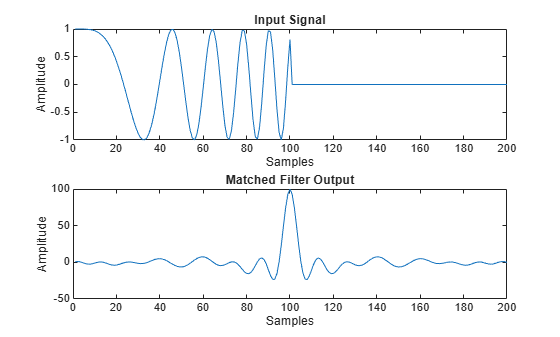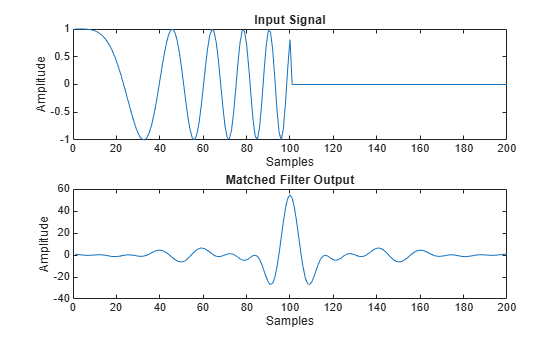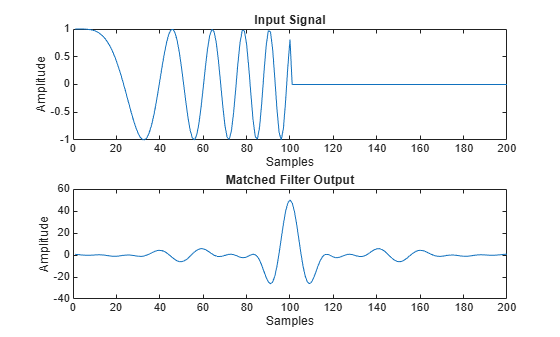# phased.MatchedFilter

Matched filter

## Description

The `MatchedFilter` object implements matched filtering of an input signal.

To compute the matched filtered signal:

1. Define and set up your matched filter. See Construction.

2. Call `step` to perform the matched filtering according to the properties of `phased.MatchedFilter`. The behavior of `step` is specific to each object in the toolbox.

Note

Starting in R2016b, instead of using the `step` method to perform the operation defined by the System object™, you can call the object with arguments, as if it were a function. For example, ```y = step(obj,x)``` and `y = obj(x)` perform equivalent operations.

## Construction

`H = phased.MatchedFilter` creates a matched filter System object, `H`. The object performs matched filtering on the input data.

`H = phased.MatchedFilter(Name,Value)` creates a matched filter object, `H`, with each specified property Name set to the specified Value. You can specify additional name-value pair arguments in any order as (`Name1`,`Value1`,...,`NameN`,`ValueN`).

## Properties

`CoefficientsSource`

Source of matched filter coefficients

Specify whether the matched filter coefficients come from the `Coefficients` property of this object or from an input argument in `step`. Values of this property are:

 `'Property'` The `Coefficients` property of this object specifies the coefficients. `'Input port'` An input argument in each invocation of `step` specifies the coefficients.

Default: `'Property'`

`Coefficients`

Matched filter coefficients

Specify the matched filter coefficients as a column vector. This property applies when you set the `CoefficientsSource` property to `'Property'`. This property is tunable.

Default: `[1;1]`

`SpectrumWindow`

Window for spectrum weighting

Specify the window used for spectrum weighting using one of `'None'`, `'Hamming'`, `'Chebyshev'`, `'Hann'`, `'Kaiser'`, `'Taylor'`, or `'Custom'`. Spectrum weighting is often used with linear FM waveform to reduce the sidelobes in the time domain. The object computes the window length internally, to match the FFT length.

Default: `'None'`

`CustomSpectrumWindow`

User-defined window for spectrum weighting

Specify the user-defined window for spectrum weighting using a function handle or a cell array. This property applies when you set the `SpectrumWindow` property to `'Custom'`.

If `CustomSpectrumWindow` is a function handle, the specified function takes the window length as the input and generates appropriate window coefficients.

If `CustomSpectrumWindow` is a cell array, then the first cell must be a function handle. The specified function takes the window length as the first input argument, with other additional input arguments if necessary, and generates appropriate window coefficients. The remaining entries in the cell array are the additional input arguments to the function, if any.

Default: `@hamming`

`SpectrumRange`

Spectrum window coverage region

Specify the spectrum region on which the spectrum window is applied as a 1-by-2 vector in the form of `[StartFrequency EndFrequency]` (in hertz). This property applies when you set the `SpectrumWindow` property to a value other than `'None'`.

Note that both `StartFrequency` and `EndFrequency` are measured in baseband. That is, they are within `[-Fs/2 Fs/2]`, where `Fs` is the sample rate that you specify in the `SampleRate` property. `StartFrequency` cannot be larger than `EndFrequency`.

Default: `[0 1e5]`

`SampleRate`

Coefficient sample rate

Specify the matched filter coefficients sample rate (in hertz) as a positive scalar. This property applies when you set the `SpectrumWindow` property to a value other than `'None'`.

Default: `1e6`

`SidelobeAttenuation`

Window sidelobe attenuation level

Specify the sidelobe attenuation level (in decibels) of a Chebyshev or Taylor window as a positive scalar. This property applies when you set the `SpectrumWindow` property to `'Chebyshev'` or `'Taylor'`.

Default: `30`

`Beta`

Kaiser window parameter

Specify the parameter that affects the Kaiser window sidelobe attenuation as a nonnegative scalar. Please refer to `kaiser` for more details. This property applies when you set the `SpectrumWindow` property to `'Kaiser'`.

Default: `0.5`

`Nbar`

Number of nearly constant sidelobes in Taylor window

Specify the number of nearly constant level sidelobes adjacent to the mainlobe in a Taylor window as a positive integer. This property applies when you set the `SpectrumWindow` property to `'Taylor'`.

Default: `4`

`GainOutputPort`

Output gain

To obtain the matched filter gain, set this property to `true` and use the corresponding output argument when invoking `step`. If you do not want to obtain the matched filter gain, set this property to `false`.

Default: `false`

## Methods

 step Perform matched filtering
Common to All System Objects
`release`

Allow System object property value changes

## Examples

collapse all

Construct a matched filter for a linear FM waveform.

```waveform = phased.LinearFMWaveform('PulseWidth',1e-4,'PRF',5e3); x = waveform(); filter = phased.MatchedFilter( ... 'Coefficients',getMatchedFilter(waveform)); y = filter(x); subplot(2,1,1),plot(real(x)) xlabel('Samples') ylabel('Amplitude') title('Input Signal') subplot(2,1,2),plot(real(y)) xlabel('Samples') ylabel('Amplitude') title('Matched Filter Output')```Apply a matched filter, using a Hamming window to do spectrum weighting.

```waveform = phased.LinearFMWaveform('PulseWidth',1e-4,'PRF',5e3); x = waveform(); filter = phased.MatchedFilter( ... 'Coefficients',getMatchedFilter(waveform), ... 'SpectrumWindow','Hamming'); y = filter(x); subplot(2,1,1) plot(real(x)) xlabel('Samples') ylabel('Amplitude') title('Input Signal') subplot(2,1,2) plot(real(y)) xlabel('Samples') ylabel('Amplitude') title('Matched Filter Output')```Apply a matched filter, using a custom Gaussian window for spectrum weighting.

```waveform = phased.LinearFMWaveform('PulseWidth',1e-4,'PRF',5e3); x = waveform(); filter = phased.MatchedFilter( ... 'Coefficients',getMatchedFilter(waveform), ... 'SpectrumWindow','Custom', ... 'CustomSpectrumWindow',{@gausswin,2.5}); y = filter(x); subplot(2,1,1) plot(real(x)) xlabel('Samples') ylabel('Amplitude') title('Input Signal') subplot(2,1,2) plot(real(y)) xlabel('Samples') ylabel('Amplitude') title('Matched Filter Output')```## Algorithms

The filtering operation uses the overlap-add method.

Spectrum weighting produces a transfer function

`$H\text{'}\left(F\right)=w\left(F\right)H\left(F\right)$`

where w(F) is the window and H(F) is the original transfer function.

For further details on matched filter theory, see or .

## References

 Richards, M. A. Fundamentals of Radar Signal Processing. New York: McGraw-Hill, 2005.

 Skolnik, M. Introduction to Radar Systems, 3rd Ed. New York: McGraw-Hill, 2001.

## Version History

Introduced in R2011a